# 深度学习与人类语言处理-语言模型

2019/04/10 10:10

### 为什么需要语言模型

HMM $\begin{equation}\mathrm{Y}^{*}=\arg \max _{\mathrm{Y}} P(X | \mathrm{Y}) P(\mathrm{Y})\end{equation}$ 在使用HMM进行语音识别时，$P(Y)$就是语言模型

LAS $\begin{equation}Y^{}=\arg \max _{Y} P(Y | X)\end{equation}$ 在端到端的深度学习模型中，是在给定$X$的情况下找到最大的$Y$,似乎没有语言模型存在的空间，但神奇的是，我们可以直接把Decoding的式子写为 $\begin{equation}Y^{}=\arg \max _{Y} P(Y | X)P(Y)\end{equation}$ 从概率的角度来看，我们很难解释这个式子的含义，乘个$P(Y)$干啥？但是为什么乘$P(Y)$有用呢，因为$P(Y|X)$和$P(Y)$的数据来源可以不同。具体来说$P(Y|X)$需要收集成对的数据，而$P(Y)$只需要收集大量的文本

google的语言识别系统使用了12500小时的声音信号，包含的词数：$1250060130$（60分钟，每分钟平均可以说130个词）,大约1亿个词

BERT数据是的语音数据的30倍，所以可以很容易的收集到大量文本数据，把$P(Y)$估的比较准，这就是为什么需要加入语言模型

### N-gram

• 怎么估计一个token sequence 的概率？

• 直观的想法，收集大量的数据，统计 $y_1,y_2,...,y_n$这个序列出现的次数，但人类的句子非常复杂，很可能句子没有出现在训练数据中，显然不能因为句子没有在训练数据中出现就认为它出现的概率是0

• N-gram语言模型：$P\left(y_{1}, y_{2}, \ldots \ldots, y_{n}\right)=P\left(y_{1} | B O S\right) P\left(y_{2} | y_{1}\right) \ldots P\left(y_{n} | y_{n-1}\right)$，就是二元语言模型，举个栗子

$P(“wreck a nice beach”) =P(wreck|START)P(a|wreck) P(nice|a)P(beach|nice)$

计算$P(beach|nice)$的概率： $P(\text { beach } | \text { nice })=\frac{C(\text {nice beach})}{C(\text {nice})}$

#### N-gram 有什么问题？

训练数据:
the dog ran...
the cat jumped...


估计新句子的概率：

$P(\text { jumped } | \text { the }, \operatorname{dog})=0$

$P(\text { ran } | \text { the }, \text { cat })=0$

#### Continuous LM

除了传统的自定义平滑规则，还有一种自动化的平滑方法，continuous LM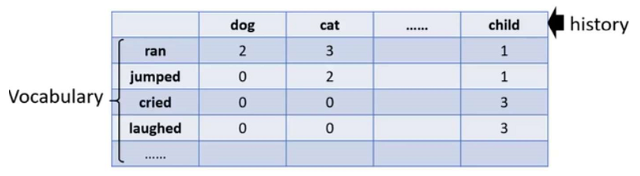表格中数字表示词数，例如$Count(ran|dog)=3,Count(ran|cat)=3$, 值为0表示训练数据中没有出现，我们想估计它的实际值，我们来看看Continuous LM怎么做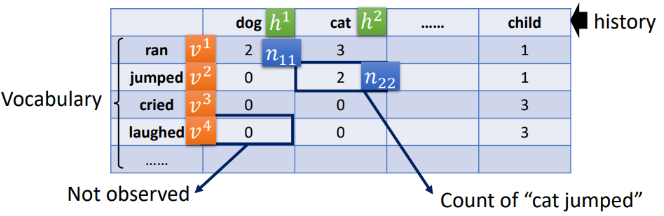• 这样做有什么好处？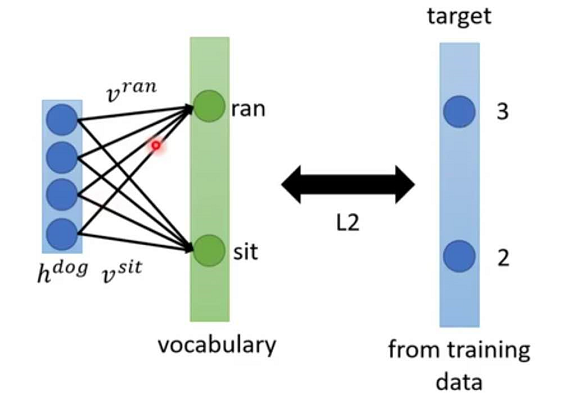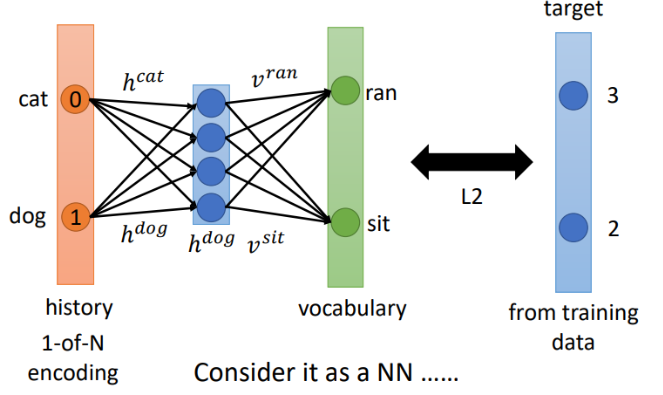### NN-based LM

• training
data: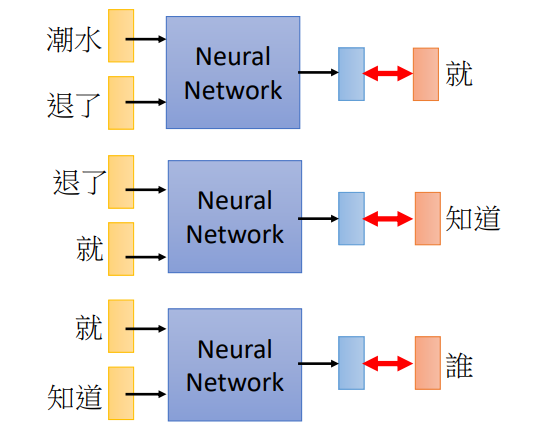$P(“wreck a nice beach”) =P(wreck|START)P(a|wreck)P(nice|a)P(beach|nice)$

$P(b|a)$：NN 预测下一个词的概率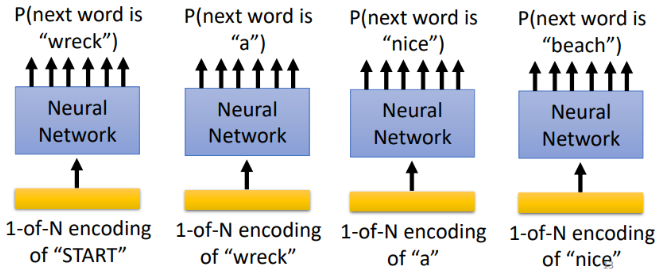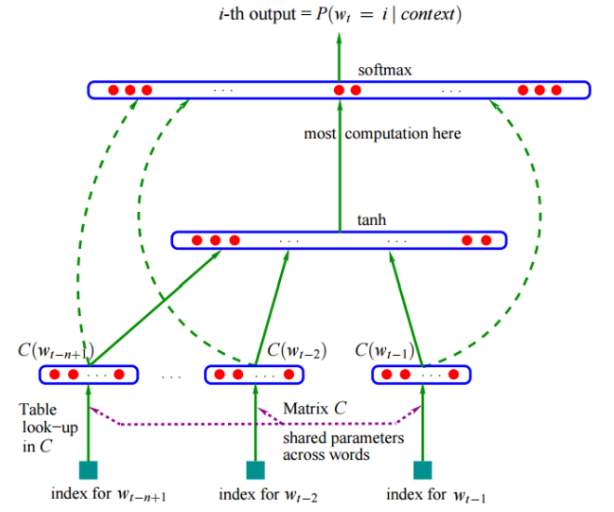### RNN-based LM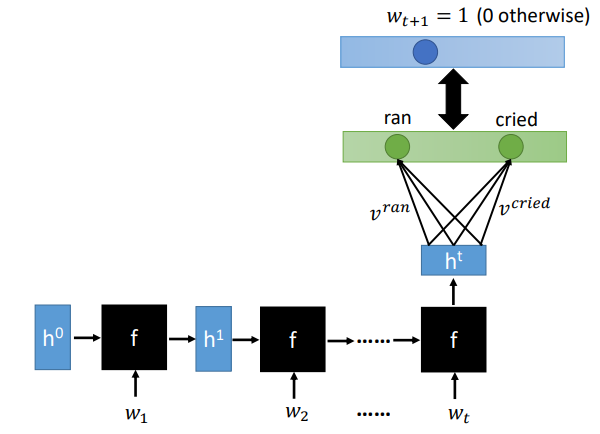###　How to use LM to improve LAS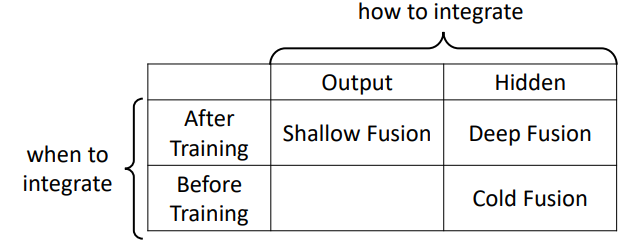• shallow fusion

分别训练LM和LAS，训练好后将各自的输出接到一起，整合

• deep fusion

分别训练LM和LAS，训练好后将各自的隐层接到一起，整合

• cold fusion

先训练LM，再将LM的隐层接到LAS进行训练

#### Shallow Fusion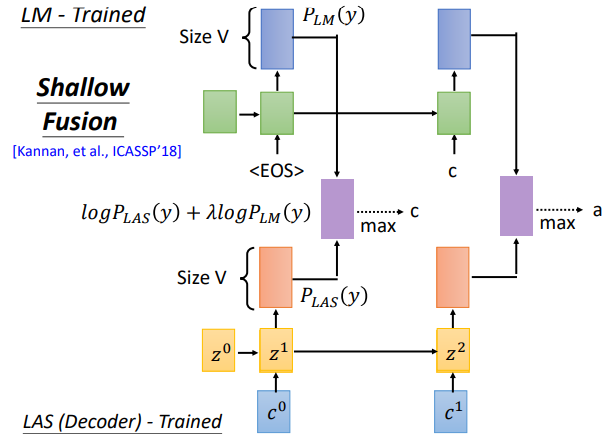### Deep Fusion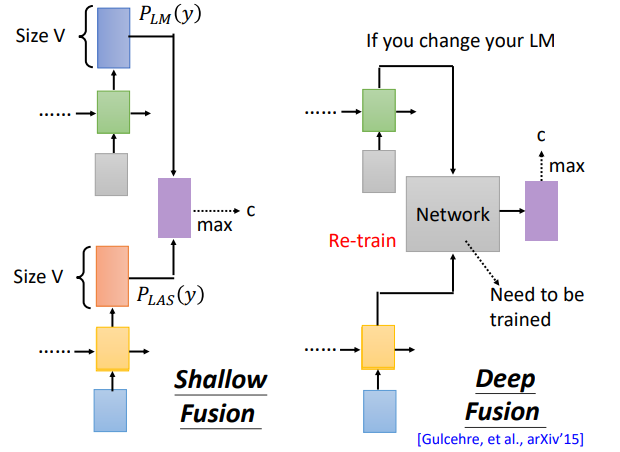### Cold Fusion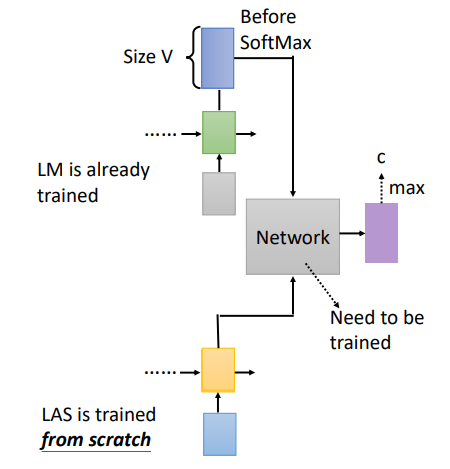references:

http://speech.ee.ntu.edu.tw/~tlkagk/courses_DLHLP20.html### 作者的其它热门文章

0
0 收藏

0 评论
0 收藏
0